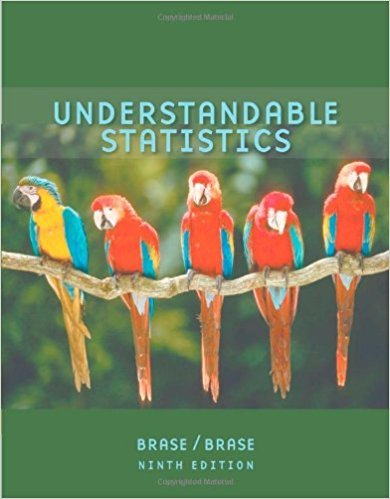×
×

# Solutions for Chapter 5.1: The Binomial Probability Distribution and Related Topics## Full solutions for Understandable Statistics | 9th Edition

ISBN: 9780618949922Solutions for Chapter 5.1: The Binomial Probability Distribution and Related Topics

Solutions for Chapter 5.1
4 5 0 236 Reviews
30
1
##### ISBN: 9780618949922

Chapter 5.1: The Binomial Probability Distribution and Related Topics includes 17 full step-by-step solutions. Understandable Statistics was written by and is associated to the ISBN: 9780618949922. Since 17 problems in chapter 5.1: The Binomial Probability Distribution and Related Topics have been answered, more than 38606 students have viewed full step-by-step solutions from this chapter. This expansive textbook survival guide covers the following chapters and their solutions. This textbook survival guide was created for the textbook: Understandable Statistics, edition: 9.

Key Statistics Terms and definitions covered in this textbook
• 2 k p - factorial experiment

A fractional factorial experiment with k factors tested in a 2 ? p fraction with all factors tested at only two levels (settings) each

• Attribute control chart

Any control chart for a discrete random variable. See Variables control chart.

• Bernoulli trials

Sequences of independent trials with only two outcomes, generally called “success” and “failure,” in which the probability of success remains constant.

• Categorical data

Data consisting of counts or observations that can be classiied into categories. The categories may be descriptive.

• Cause-and-effect diagram

A chart used to organize the various potential causes of a problem. Also called a ishbone diagram.

• Comparative experiment

An experiment in which the treatments (experimental conditions) that are to be studied are included in the experiment. The data from the experiment are used to evaluate the treatments.

• Conditional probability

The probability of an event given that the random experiment produces an outcome in another event.

• Control chart

A graphical display used to monitor a process. It usually consists of a horizontal center line corresponding to the in-control value of the parameter that is being monitored and lower and upper control limits. The control limits are determined by statistical criteria and are not arbitrary, nor are they related to speciication limits. If sample points fall within the control limits, the process is said to be in-control, or free from assignable causes. Points beyond the control limits indicate an out-of-control process; that is, assignable causes are likely present. This signals the need to ind and remove the assignable causes.

• Counting techniques

Formulas used to determine the number of elements in sample spaces and events.

• Crossed factors

Another name for factors that are arranged in a factorial experiment.

• Decision interval

A parameter in a tabular CUSUM algorithm that is determined from a trade-off between false alarms and the detection of assignable causes.

• Erlang random variable

A continuous random variable that is the sum of a ixed number of independent, exponential random variables.

• Error propagation

An analysis of how the variance of the random variable that represents that output of a system depends on the variances of the inputs. A formula exists when the output is a linear function of the inputs and the formula is simpliied if the inputs are assumed to be independent.

• Exhaustive

A property of a collection of events that indicates that their union equals the sample space.

• F-test

Any test of signiicance involving the F distribution. The most common F-tests are (1) testing hypotheses about the variances or standard deviations of two independent normal distributions, (2) testing hypotheses about treatment means or variance components in the analysis of variance, and (3) testing signiicance of regression or tests on subsets of parameters in a regression model.

• First-order model

A model that contains only irstorder terms. For example, the irst-order response surface model in two variables is y xx = + ?? ? ? 0 11 2 2 + + . A irst-order model is also called a main effects model

• Fractional factorial experiment

A type of factorial experiment in which not all possible treatment combinations are run. This is usually done to reduce the size of an experiment with several factors.

• Frequency distribution

An arrangement of the frequencies of observations in a sample or population according to the values that the observations take on

• Gamma random variable

A random variable that generalizes an Erlang random variable to noninteger values of the parameter r

• Harmonic mean

The harmonic mean of a set of data values is the reciprocal of the arithmetic mean of the reciprocals of the data values; that is, h n x i n i = ? ? ? ? ? = ? ? 1 1 1 1 g .

×